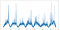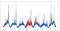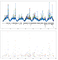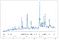Ro spends a significant amount of money on commercials, especially on TV. These commercials tend to drive a high amount of traffic within a relatively concentrated period of time. They also drive a long tail of site traffic over the next day/days/weeks.

Ro does in-house attribution modeling for commercials to cross-reference the analytics we get back from our vendors and make sure the top level numbers are accurate.

One of the first steps for TV commercial site traffic attribution is to identify spikes in traffic. This article outlines the first iteration of an algorithm we’ve previously used to identify said spikes.

The most advanced mathematical function used here is convolution, used for signal smoothing. You can definitely employ this algorithm without understanding the mechanics of how convolution works.

# The Starting Anomaly Detection Algorithm: Z Score Thresholding

We began with the “smoothed z score algorithm” (see also: python implementation). With a name far more complex than its implementation, this algorithm boils down to 25 lines of code and is based only on the concepts of average and standard deviation. The trickiest aspect is tuning the 3 parameters for optimal performance.

At any position in the signal, the smoothed z score algorithm essentially computes a trailing average of and a trailing standard deviation of the preceding window of data points specified by the lag parameter. If a point within the lag window is considered to be part of an identified spike/anomaly, this point is not given full value, but rather weighted only as much as the influence parameter. If the point at the current position has a value outside of `​​​​trailing average` +/- ( `threshold` * `trailing standard deviation`) then it is considered to be part of an anomaly and marked as such.

# Creating A More Robust Thresholding Algorithm

The Z Score algorithm is fairly robust for flat signals that experience intermittent spikes. However, our traffic signals have a non-constant base (i.e. a daily cycle):Example Ro traffic signal during a week with commercials (7 days); y axis is a measure of session traffic magnitude, x axis is time — we chose a granularity of 1 x-axis step = 3 minutes of time span

Attempting to use the basic Z Score algorithm results in either oversensitivity or undersensitivity (depending on how the parameters are tuned), with little middle ground.The best possible tuning of the forward pass still has serious issues, especially for day 4

# Step1: Apply the Z Score Algorithm in Reverse

The smoothed z score algorithm moves from left to right. If we apply the algorithm from right to left, we will get a different result which we can then compare against the original version.

Here is what this looks like in python:

`def thresholding_algo_reversed(y, lag, threshold, influence):    signals = np.zeros(len(y))    filteredY = np.array(y)    avgFilter = *len(y)    stdFilter = *len(y)    avgFilter[-lag] = np.mean(y[-lag:])    stdFilter[-lag] = np.std(y[-lag:])    for i in reversed(range(0, len(y)-lag)):        if abs(y[i] - avgFilter[i+1]) > threshold * stdFilter [i+1]:            if y[i] > avgFilter[i+1]:                signals[i] = 1            else:                signals[i] = 0            filteredY[i] = influence * y[i] + (1 - influence) * filteredY[i+1]            avgFilter[i] = np.mean(filteredY[i:(i+lag)])            stdFilter[i] = np.std(filteredY[i:(i+lag)])        else:            signals[i] = 0            filteredY[i] = y[i]            avgFilter[i] = np.mean(filteredY[i:(i+lag)])            stdFilter[i] = np.std(filteredY[i:(i+lag)])    return dict(signals = np.asarray(signals),        avgFilter = np.asarray(avgFilter),        stdFilter = np.asarray(stdFilter))`

# Step 2: Compute a New Baseline Signal From the Forward and Reverse Z Score Algorithm Results

The inputs here from previous steps are:

• the forward pass moving average, computed previously
• the reverse pass moving average, computed previously
• the list of identified anomaly points from the forward pass, computed previously
• the list of identified anomaly points from the reverse pass, computed previously

New parameters introduced are:

• `multiple_cutoff`
• `yes_weight_within_cutoff`
• `yes_weight_outside_cutoff`

For every point in the original signal:

Here’s what this looks like as python code:

`#initialize arrays of zerosstd_combined = np.zeros(len(std_forward))avg_combined = np.zeros(len(avg_forward))#new parametersmultiple_cutoff = 1.5yes_weight_within_cutoff = .8yes_weight_outside_cutoff = .05for i in range(0,len(avg_forward)):    if avg_forward[i] == 0:        avg_combined[i] = avg_reversed[i]        std_combined[i] = std_reversed[i]    elif avg_reversed[i] == 0:        avg_combined[i] = avg_forward[i]        std_combined[i] = std_forward[i]    elif signal_forward[i] == signal_reversed[i]:        avg_combined[i] = (avg_forward[i] + avg_reversed[i])/2        std_combined[i] = (std_forward[i] + std_reversed[i])/2    elif signal_forward[i]:        if 1.0 * avg_reversed[i]/avg_forward[i] < multiple_cutoff:            yes_weight = yes_weight_within_cutoff        else:            yes_weight = yes_weight_outside_cutoff        avg_combined[i] = (yes_weight) * avg_forward[i] + (1-yes_weight) * avg_reversed[i]        std_combined[i] = (yes_weight) * std_forward[i] + (1-yes_weight) * std_reversed[i]    elif signal_reversed[i]:        if 1.0 * avg_forward[i]/avg_reversed[i] < multiple_cutoff:            yes_weight = yes_weight_within_cutoff        else:            yes_weight = yes_weight_outside_cutoff    avg_combined[i] = (1-yes_weight) * avg_forward[i] + (yes_weight) * avg_reversed[i]    std_combined[i] = (1-yes_weight) * std_forward[i] + (yes_weight) * std_reversed[i]    else:        print("unhandled condition")`

# Step 3: Convolve the New Baseline Signal to Smooth It

Our use case for convolution is essentially to smooth our new signal in a way that is better than a simple trailing average (which only incorporate data to the left of the point being smoothed). Here we’ve applied a short horizontal line as the convolution signal — the sum of the values of each of the points in the signal is 1. For our use case the convolution length (which can be thought of as another tunable parameter) worked well as 40 (meaning that we’re smoothing every point with 20 points from the left and 20 points from the right).

In python, convolving can be done with the numpy library:

`avg_convolve_length = 40.0avg_convolve_weights = np.ones(int(avg_convolve_length)) / avg_convolve_lengthstd_convolve_length = 2*avg_convolve_lengthstd_convolve_weights = np.ones(int(std_convolve_length)) / std_convolve_lengthavg_combined = np.convolve(avg_combined,avg_convolve_weights,'same')std_combined = np.convolve(std_combined,std_convolve_weights,'same')`

The convolution is applied to both the new baseline and the new standard deviation signals.

# Step 4: Re-identify Anomaly Points Using the New Smoothed Baseline

This is quite basic. Given our new baseline signal and standard deviation signal, any points in the actual signal that are `threshold` standard deviations above the baseline are considered to be part of an anomaly spike.

The logic to do so is here:

`neighbor_threshold = .5neighbor_in_between_threshold = -.5neighbor_distance_range = 2for index in signal_combined.nonzero():    for distance in range(1, neighbor_distance_range+1):        if index-distance >= 0 and \            numsessions_array[index-distance] != 1 and \            numsessions_array[index-distance+1] > avg_combined[index-distance+1] +            neighbor_in_between_threshold * std_combined[index-distance+1] and \            numsessions_array[index-distance] >= avg_combined[index-distance] +            neighbor_threshold * std_combined[index-distance]:                signal_combined[index-distance] = 1        if index+distance < len(signal_combined) and \            numsessions_array[index+distance] != 1 and \            numsessions_array[index+distance-1] > avg_combined[index+distance-1] +            neighbor_in_between_threshold * std_combined[index+distance-1] and \            numsessions_array[index+distance] >= avg_combined[index+distance] +            neighbor_threshold * std_combined[index+distance]:            signal_combined[index+distance] = 1`

# Grouping Anomaly Points Into Spikes, Filtering Spikes Using TV Logs

The new input here is:

• the tv commercial log

How you proceed at this point is dependent on the desired granularity of attribution. On the simplest level, we just want to make sure that identified anomalies are within a reasonable range of an aired commercial — an anomaly that is 1 hr before/after the nearest commercial airing is in all likelihood not commercial related.

In our use case we were most interested in this analysis to get a top level figure of traffic/conversions that came in from television every week. This top level figure was compared to what our TV buying agency was reporting in order to give us confidence in their numbers. Once we had established confidence in this top level number we were happy to let them break down which channels/spots were the most efficient.

# Splitting Anomaly Groups Into Spikes

Depending on how your anomalies look, how concurrent your tv commercials are, and what level of granularity you are aiming for you may also need to consider the need for splitting certain anomalies into multiple, overlapping spikes.

The simplest way to split up an anomaly group is to look for local minima (i.e. where the first derivative is 0) — these mark points in time where descending traffic from an earlier spike is overtaking by increasing traffic from a subsequent spike.

Here is a python implementation:

`import more_itertools as mitsignal_non_zeros_indices = signal_combined.nonzero()grouped_before_split = [tuple(group) for group in mit.consecutive_groups(signal_non_zeros_indices)]def map_delta(delta):    if delta < 0:        return -1    elif delta == 0:        return 0    else:        return 1def split_group(group_tuple, sessions_array):    if(len(group_tuple) == 1):        return [group_tuple]    split_local_indices = [group_tuple, group_tuple[-1]+1]    for local_index in range(1,len(group_tuple)-1):        pre_delta_map = map_delta(sessions_array[group_tuple[local_index]] - \            sessions_array[group_tuple[local_index-1]])        post_delta_map = map_delta(sessions_array[group_tuple[local_index+1]] - \            sessions_array[group_tuple[local_index]])        if (pre_delta_map * post_delta_map in [-1,0]):            if post_delta_map == 1:                split_local_indices.append(group_tuple[local_index])    split_local_indices = sorted(split_local_indices)    tuple_groups = []    for i in range(0,len(split_local_indices)-1): tuple_groups.append(tuple(range(split_local_indices[i],split_local_indices[i+1])))       return tuple_groupsgrouped = []for group_tuple in grouped_before_split:    grouped = grouped + split_group(group_tuple, numsessions_array)cutoff_added_sessions = 0spikes_dict = {}for group in grouped:    above_ma = 0    for index in group:        above_ma += numsessions_array[index] - avg_combined[index]    if above_ma >= cutoff_added_sessions:        spikes_dict[group] = round(above_ma,1)grouped=spikes_dict.keys()`Top: Signal anomalies divided into distinct spikes — consecutive spikes have different colors; the black dots on the bottom represent tv spots airing — the majority of commercials were not run exactly concurrently with each other, but in some cases there were 2 (and even 3 and 4) commercials running concurrently | Bottom: Each spike is given an overall magnitude, equal to the total number of sessions attributed to the spike above the baseline signal.A zoomed in version of segmenting anomaly groups into distinct spikes, each with a different color; the baseline signal and standard deviation signals are illustrated with dashes; the black dots represent tv commercials, and a black dot on the lower tier represents two commercials running within the same time window

It is important to note that these local minima don’t identify the divisions between spikes, which are in fact overlapping; rather, they identify the point in time where the later spike “overpowers” the earlier one.

# Extension: Granular Value Attribution for Concurrent Commercials

If you are

1. looking to assign specific values to individual commercials and
2. are running lots of commercials within minutes/seconds of each other and can’t simply match every commercial with the nearest spike

you will need a more sophisticated solution to commercial<>spike matching. One contender here is constrained optimization (see also: constrained optimization in python).

The objective would be:

• maximize `the number of spikes attributed to commercial airings` + `the number of commercial airings attributed to a spike`

Constraints would include:

• a spike must begin within a defined period of time from the nearest commercial airing (ex: 3 minutes)
• a single commercial can only yield a single spike, though a single spike may be the result of multiple commercials

## Ro Data Team Blog

#### Ro Data Team Blog: data analytics, data engineering, data science